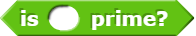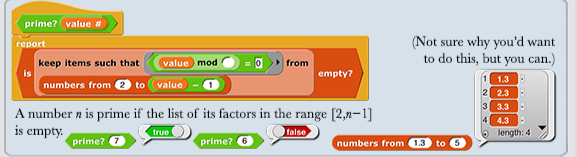# How do you check if a number is prime?

Edit: I just want to make a blockthat checks, as quickly as possible, if the number is prime.

Loop from $$2$$ to $$\lfloor \sqrt{n} \rfloor$$ (floor of the square root of n, the number if that's not cleaer) and check whether or not $$n$$ is divisible by that number. If it's not divisible by any number in that range then it's prime

Let's say. Each prime number (n) when I divide n by 2 I will have after the decimal point 3333 or 66666666667 and so on.

n₁:3=n₂,33333

Where n₂ is a integer

But I don't know this always works right :-/

Examples

19:3= 6, 3333 ≈ 6,33
67:3 = 22,3333 ≈ 22,33
97:3 = 32,33333 ≈ 32,333
5:3 ≈ 1,67

Counterargument: 1 isn't prime but 1/3 = 0.333333333333333...
Counterargument 2: 25 isn't prime but 25/3 = 8 1/3 = 8.333333333333333...

Unfortunately :-/

I made a detector of prime numbers. Check- maybe work right...

That will never give a false negative, i.e., if it says the number isn't prime, then the number isn't prime, but it'll give a lot of false positives:
1
4
9
12
16
.
.
.

Interestingly enough, those numbers seem to follow +3 then +4 then +3 then +4 etc.

Somehow implement the sieve of Eratosthene.  [ O(log(log(n)) vs O(n) ] Sieve of Eratosthenes - Wikipedia

This is the answer, you guys.

Earlier it worked good.

Edit: Now should work right. I forgot the ≥ character and unnecessarily I add a a-2